Скачать презентацию ITEC 2620 M Introduction to Data Structures Instructor

a263f5c224bd8a3c29640635e757ac2e.ppt

• Количество слайдов: 23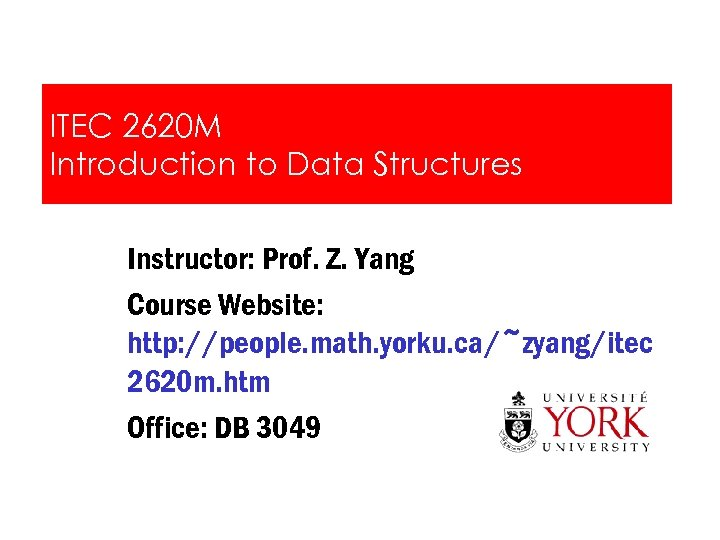ITEC 2620 M Introduction to Data Structures Instructor: Prof. Z. Yang Course Website: http: //people. math. yorku. ca/~zyang/itec 2620 m. htm Office: DB 3049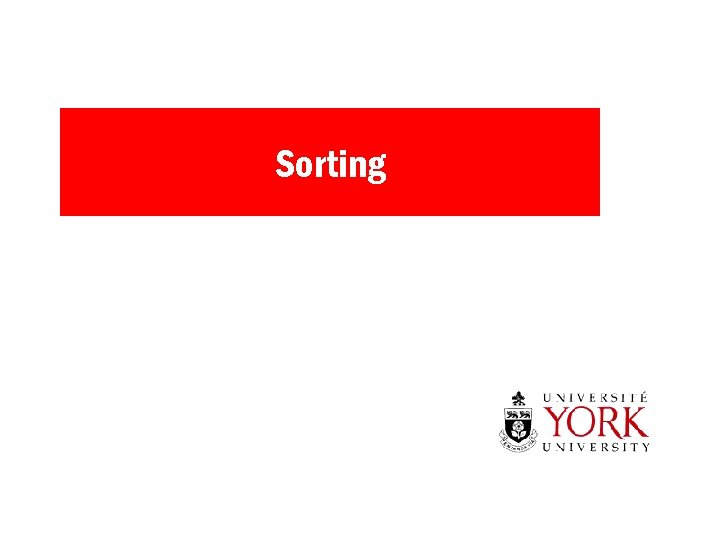Sorting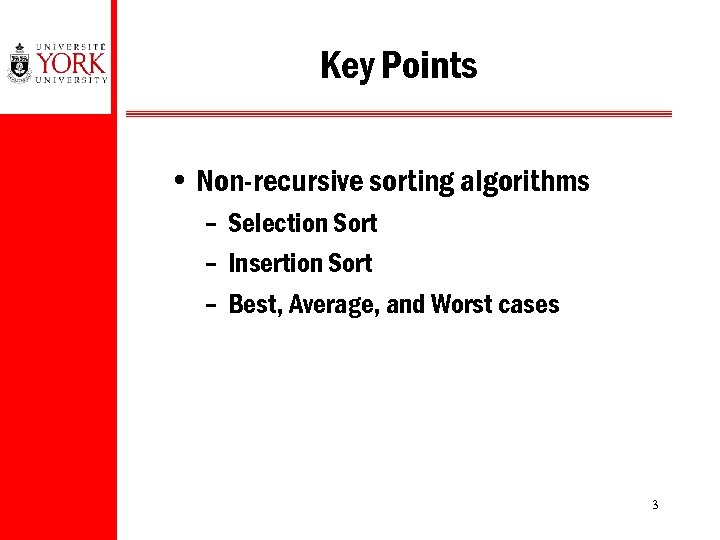Key Points • Non-recursive sorting algorithms – Selection Sort – Insertion Sort – Best, Average, and Worst cases 3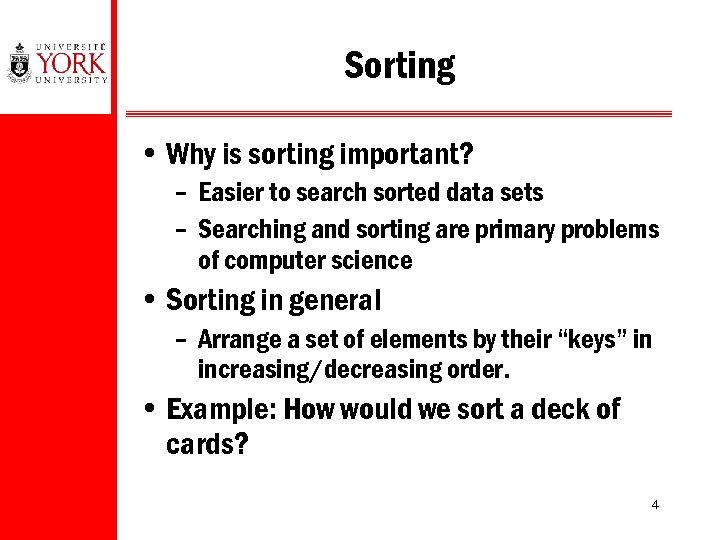Sorting • Why is sorting important? – Easier to search sorted data sets – Searching and sorting are primary problems of computer science • Sorting in general – Arrange a set of elements by their “keys” in increasing/decreasing order. • Example: How would we sort a deck of cards? 4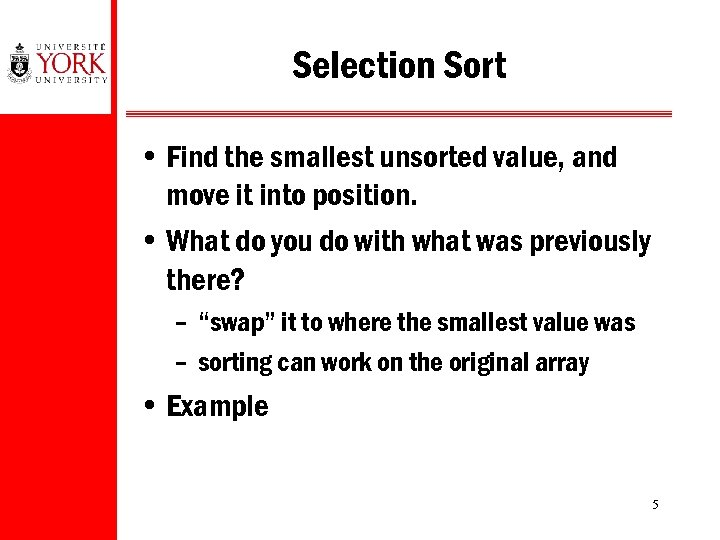Selection Sort • Find the smallest unsorted value, and move it into position. • What do you do with what was previously there? – “swap” it to where the smallest value was – sorting can work on the original array • Example 5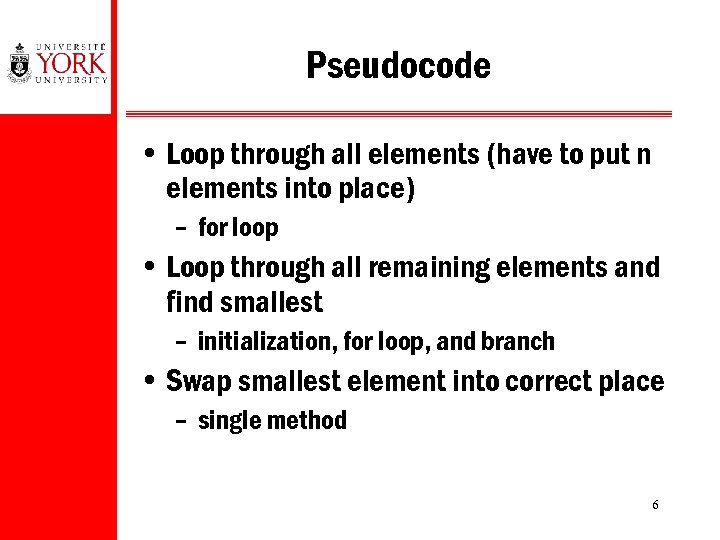Pseudocode • Loop through all elements (have to put n elements into place) – for loop • Loop through all remaining elements and find smallest – initialization, for loop, and branch • Swap smallest element into correct place – single method 6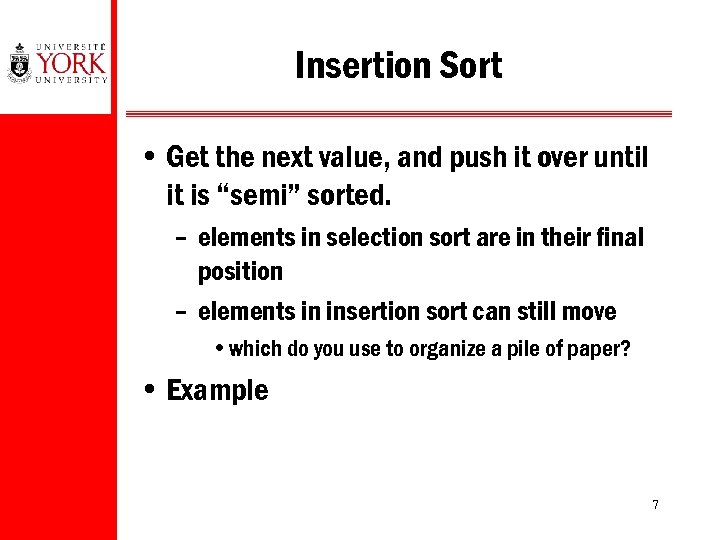Insertion Sort • Get the next value, and push it over until it is “semi” sorted. – elements in selection sort are in their final position – elements in insertion sort can still move • which do you use to organize a pile of paper? • Example 7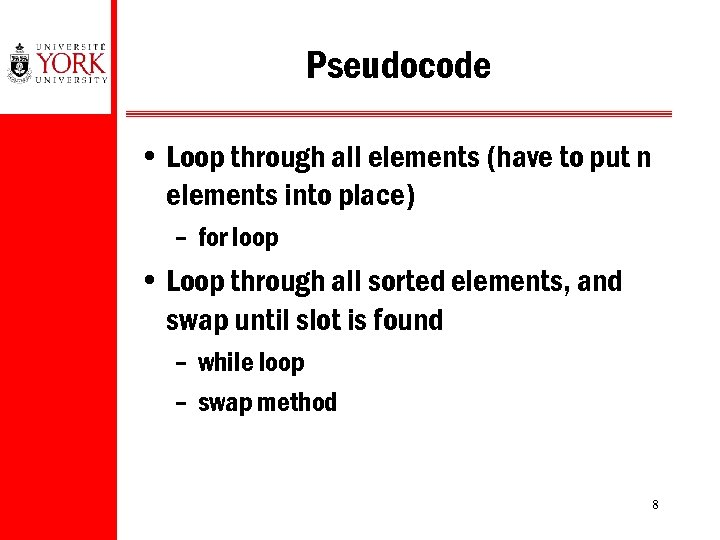Pseudocode • Loop through all elements (have to put n elements into place) – for loop • Loop through all sorted elements, and swap until slot is found – while loop – swap method 8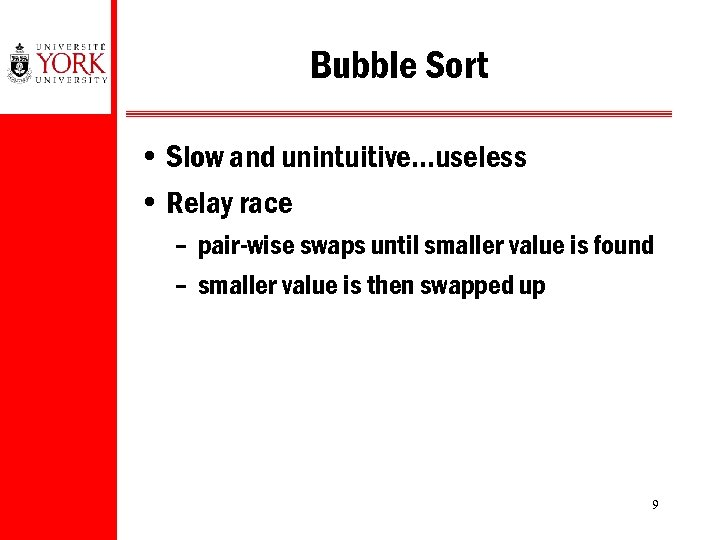Bubble Sort • Slow and unintuitive…useless • Relay race – pair-wise swaps until smaller value is found – smaller value is then swapped up 9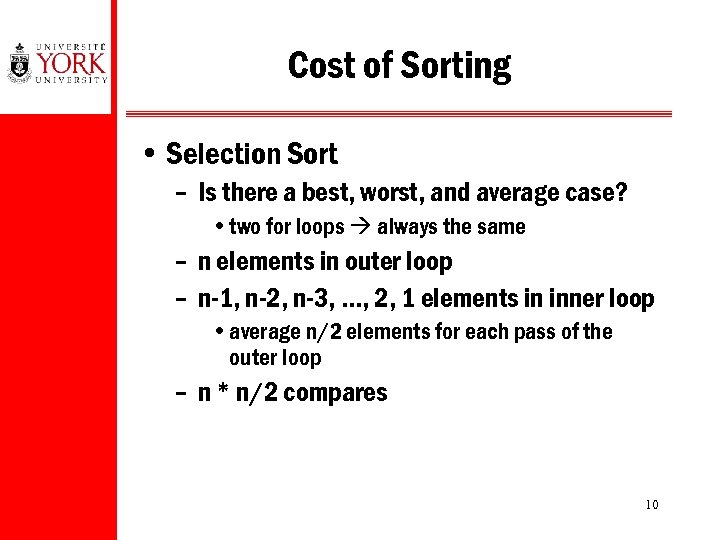Cost of Sorting • Selection Sort – Is there a best, worst, and average case? • two for loops always the same – n elements in outer loop – n-1, n-2, n-3, …, 2, 1 elements in inner loop • average n/2 elements for each pass of the outer loop – n * n/2 compares 10Cost of Sorting (Cont’d) • Insertion Sort – Worst – same as selection sort, next element swaps till end • n * n/2 compares – Best – next element is already sorted, no swaps • n * 1 compares – Average – linear search, 50% of values n/4 • n * n/4 compares 11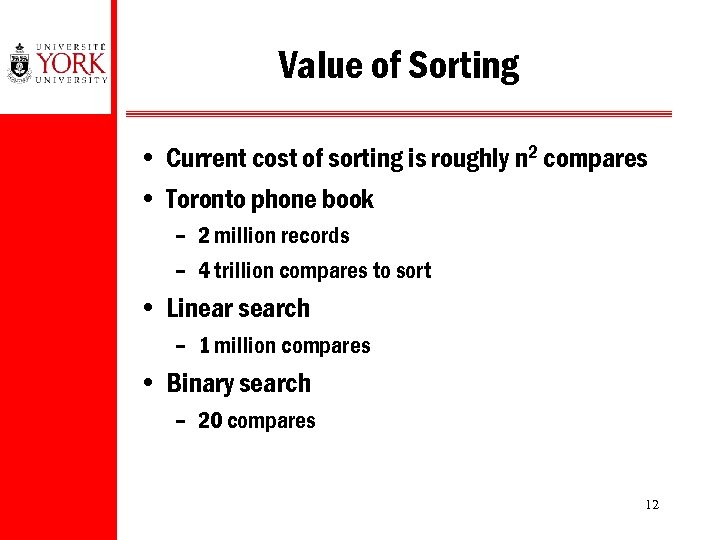Value of Sorting • Current cost of sorting is roughly n 2 compares • Toronto phone book – 2 million records – 4 trillion compares to sort • Linear search – 1 million compares • Binary search – 20 compares 12Trade-Offs • Write a method, or re-implement each time? • Buy a parking pass, or pay cash each time? • Sort in advance, or do linear search each time? • Trade-offs are an important part of program design – which component should you optimize? – is the cost of optimization worth the savings? 13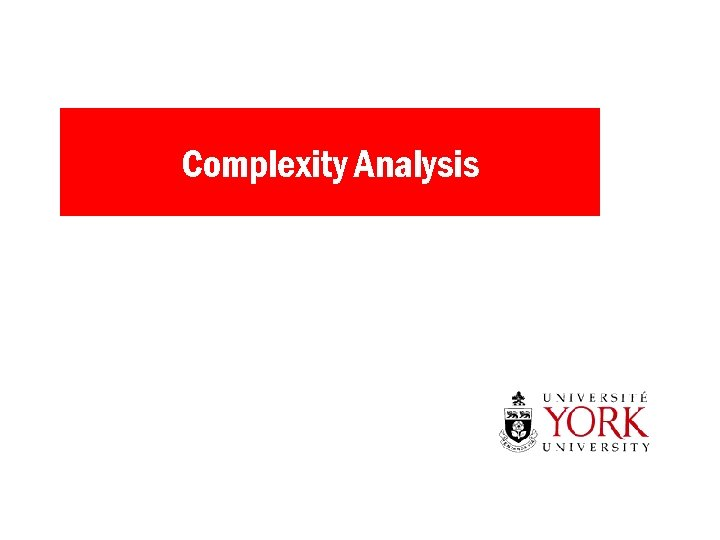Complexity Analysis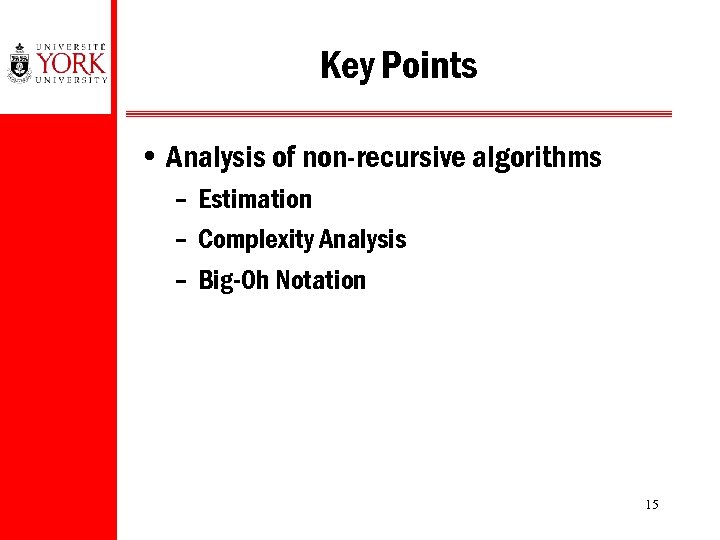Key Points • Analysis of non-recursive algorithms – Estimation – Complexity Analysis – Big-Oh Notation 15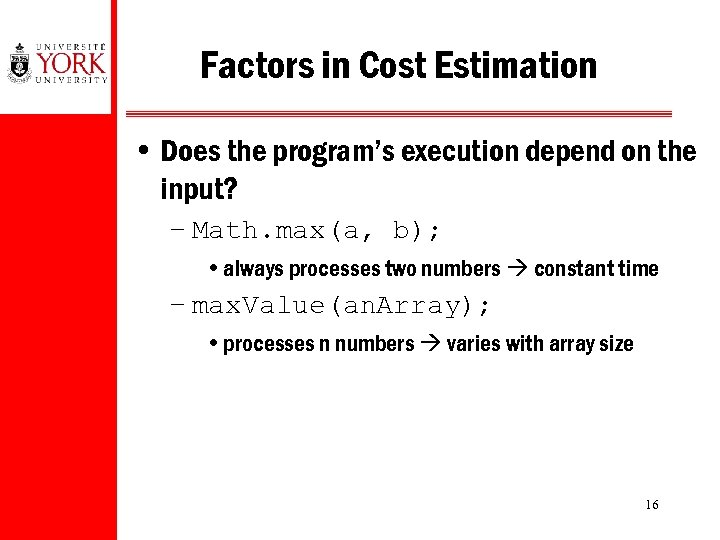Factors in Cost Estimation • Does the program’s execution depend on the input? – Math. max(a, b); • always processes two numbers constant time – max. Value(an. Array); • processes n numbers varies with array size 16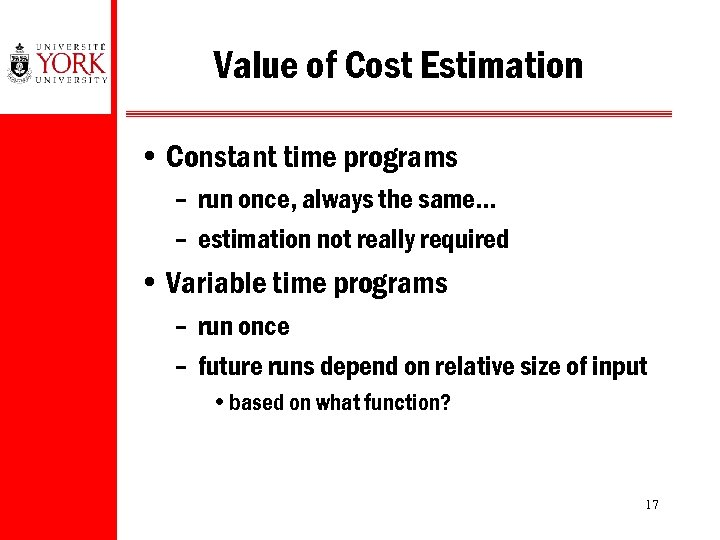Value of Cost Estimation • Constant time programs – run once, always the same… – estimation not really required • Variable time programs – run once – future runs depend on relative size of input • based on what function? 17Cost Analysis • Consider the following code: sum = 0; for (i=1; i<=n; i++) for (j=1; j<=n; j++) sum++; • It takes longer when n is larger. 18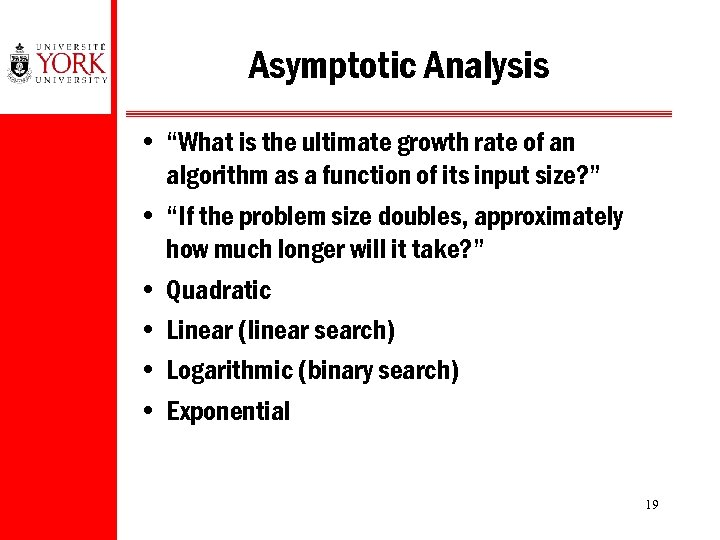Asymptotic Analysis • “What is the ultimate growth rate of an algorithm as a function of its input size? ” • “If the problem size doubles, approximately how much longer will it take? ” • Quadratic • Linear (linear search) • Logarithmic (binary search) • Exponential 19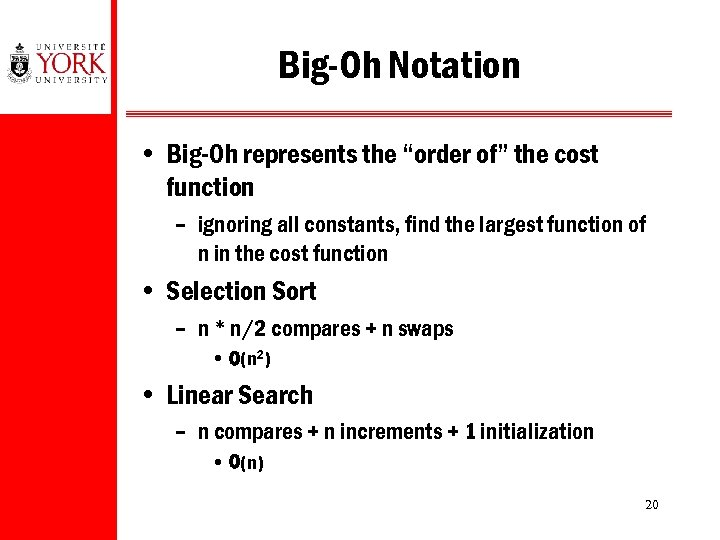Big-Oh Notation • Big-Oh represents the “order of” the cost function – ignoring all constants, find the largest function of n in the cost function • Selection Sort – n * n/2 compares + n swaps • O(n 2) • Linear Search – n compares + n increments + 1 initialization • O(n) 20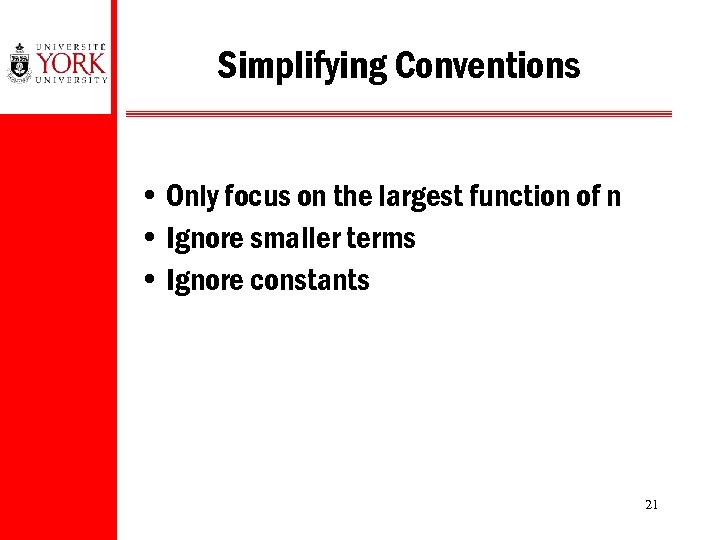Simplifying Conventions • Only focus on the largest function of n • Ignore smaller terms • Ignore constants 21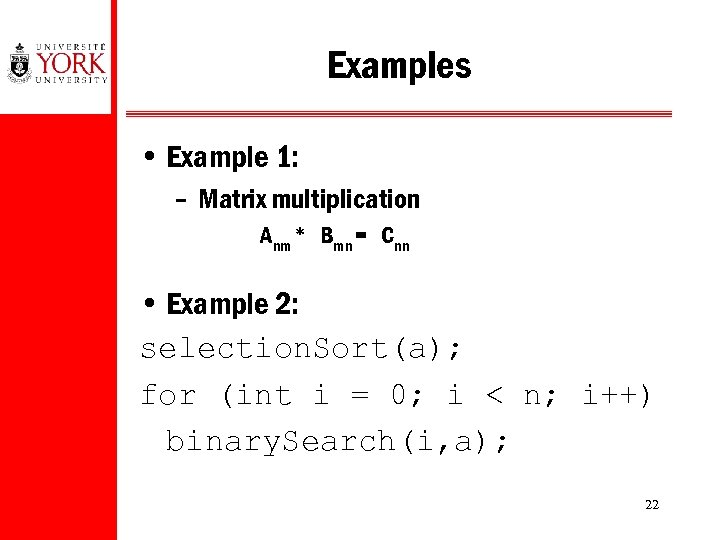Examples • Example 1: – Matrix multiplication Anm * Bmn = Cnn • Example 2: selection. Sort(a); for (int i = 0; i < n; i++) binary. Search(i, a); 22Trade-Offs and Limitations • “What is the dominant term in the cost function? ” – What if the constant term is larger than n? • What happens if both algorithms have the same complexity? – Selection sort and Insertion sort are both O(n 2) • Constants can matter – Same complexity (obvious) and different complexity (problem size) 23